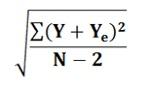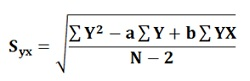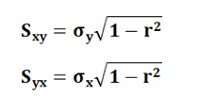Skip to content

# Standard error of estimate

The Standard Error of Estimate is the measure of variation of an observation made around the computed regression line. Simply, it is used to check the accuracy of predictions made with the regression line.

Likewise, a standard deviation which measures the variation in the set of data from its mean, the standard error of estimate also measures the variation in the actual values of Y from the computed values of Y (predicted) on the regression line. It is computed as a standard deviation, and here the deviations are the vertical distance of every dot from the line of average relationship.

The deviation of each dot from the regression line is expressed as Y-Ye, thus the square root of mean of standard deviation is:Y = actual values
Ye= estimated values

This formula is not convenient as it requires to calculate the estimated value of Y i.e. Ye. Thus, more convenient and easy formula is given below:Similarly, the value of Sxy can be calculated by using the following formula:Once these values are calculated, the standard error of estimate can be calculated easily by using the following formulae:The smaller the value of a standard error of estimate the closer are the dots to the regression line and better is the estimate based on the equation of the line. If the standard error is zero, then there is no variation corresponding to the computed line and the correlation will be perfect.

Thus, the standard error of estimate measures the accuracy of the estimated figures, i.e. it is possible to ascertain the goodness and representativeness of the regression line as a description of the average relationship between the two series.

### 1 Comment »

error: Content is protected !!